Completely Solved C, C++ Programs Assignment.Quick Search Database Videos Tutorials Ebooks Forums FAQ Aboutus Household Industrial Manufacturing Service Shopping Transportation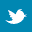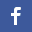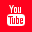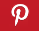### C program to implement Priority Queue using heap

 Filed Under: C Assignments

Program Statement :
Write a C program to implement Priority Queue using heap.

Theory :
A Priority Queue is an abstract data type to efficiently support finding an item with the highest priority across a series of operations. The basic operations are :
1. Insert,
2. Find - minimum (or maximum), and
3. Delete-minimum (or maximum).
Some implementations also efficiently support, join two priority queues (meld), delete an arbitrary item, and increase the priority of a item (decrease-key).
A heap is a specialized tree-based data structure that satisfies the heap property : if B is a child node of A, then key(A) ≥ key(B). This implies that an element with the greatest key is always in the root node, and so such a heap is sometimes called a max-heap. (Alternatively, if the comparison is reversed, the smallest element is always in the root node, which results in a min-heap). The several variants of heaps are the prototypical most efficient implementations of the abstract data type priority queues. Priority queues are useful in many applications. In particular, heaps are crucial in several efficient graph algorithms.
Heaps are usually implemented in an array, and don't require pointers between elements.
The operations commonly performed with a heap are :
 delete-max or delete-min : removing the root node of a max or min heap, respectively.
 increase-key or decrease-key : updating a key within a max or min heap, respectively.
 insert : adding a new key to the heap.
 merge : joining two heaps to form a valid new heap containing all the elements of both.

Algorithm :
&#61548; Insertion.
/*This algorithm inserts an element in the queue*/
Algo_insert(a[],int heapsize,data,lb)
{
if(heapsize==MAX)/*MAX denotes maximum size of queue*/
{
printf(Queue Is Full!);
exit from program;
}
i=lb+heapsize;
a[i]=data;
while(i>lb AND a[p=parent(i)]<a[i])
{
swap(a[p],a[i]);
i=p;
}
}
&#61548; Deletion.

/*This function deletes an element from the queue*/
int del_hi_priori(a[], heapsize, lb)
{
if(heapsize==1)
{
printf(Queue Is Empty!);
exit from program;
}
t=a[lb];
swap(a[lb],a[heapsize-1]);
i=lb;
heapsize=heapsize-1;
while(1)
{
if((l=left(i))>=heapsize)
exit;
if((r=right(i))>=heapsize)
max_child=l;
else
max_child=(a[l]>a[r])?l:r;
if(a[i]>=a[max_child])
exit;
swap(&a[i],&a[max_child]);
i=max_child;
}
return t;
}

Program listing :
/* C program to implement Priority Queue using heap */
#include<stdio.h>
#include<math.h>
#define MAX 100/*Declaring the maximum size of the queue*/
void swap(int*,int*);
main()
{
int choice,num,n,a[MAX],data,s;
void display(int[],int);
void insert(int[],int,int,int);
int del_hi_priori(int[],int,int);
n=0;/*Represents number of nodes in the queue*/
int lb=0;/*Lower bound of the array is initialized to 0*/
while(1)
{
printf("n1.Insert.n");
printf("2.Delete.n");
printf("3.Display.n");
printf("4.Quit.n");
scanf("%d",&choice);
switch(choice)
{
case 1:/*choice to accept an elemnt and insert it in the queue*/
printf("Enter data to be inserted : ");
scanf("%d",&data);
insert(a,n,data,lb);
n++;
break;
case 2:
s=del_hi_priori(a,n+1,lb);
if(s!=0)
printf("nThe deleted value is : %d n",s);
if(n>0)
n--;
break;
case 3:/*choice to display the elements of the queue*/
printf("n");
display(a,n);
break;
case 4:/*choice to exit from the program*/
return;
default:
printf("Invalid choice.n");
}
printf("nn");
}
}
/*This function inserts an element in the queue*/
void insert(int a[],int heapsize,int data,int lb)
{
int i,p;
int parent(int);
if(heapsize==MAX)
{
printf("Queue Is Full!!n");
return;
}
i=lb+heapsize;
a[i]=data;
while(i>lb&&a[p=parent(i)]<a[i])
{
swap(&a[p],&a[i]);
i=p;
}
}
/*This function deletes an element from the queue*/
int del_hi_priori(int a[],int heapsize,int lb)
{
int data,i,l,r,max_child,t;
int left(int);
int right(int);
if(heapsize==1)
{
printf("Queue Is Empty!!n");
return 0;
}
t=a[lb];
swap(&a[lb],&a[heapsize-1]);
i=lb;
heapsize--;
while(1)
{
if((l=left(i))>=heapsize)
break;
if((r=right(i))>=heapsize)
max_child=l;
else
max_child=(a[l]>a[r])?l:r;
if(a[i]>=a[max_child])
break;
swap(&a[i],&a[max_child]);
i=max_child;
}
return t;
}
/*Returns parent index*/
int parent(int i)
{
float p;
p=((float)i/2.0)-1.0;
return ceil(p);
}
/*Returns leftchild index*/
int left(int i)
{
return 2*i+1;
}
/*Returns rightchild index*/
int right(int i)
{
return 2*i+2;
}
/*This function displays the queue*/
void display(int a[],int n)
{
int i;
if(n==0)
{
printf("Queue Is Empty!!n");
return;
}
for(i=0;i<n;i++)
printf("%d ",a[i]);
printf("n");
}
/*This function is used to swap two elements*/
void swap(int*p,int*q)
{
int temp;
temp=*p;
*p=*q;
*q=temp;
}

Output :

1.Insert.
2.Delete.
3.Display.
4.Quit.
Enter data to be inserted : 52

1.Insert.
2.Delete.
3.Display.
4.Quit.
Enter data to be inserted : 63

1.Insert.
2.Delete.
3.Display.
4.Quit.
Enter data to be inserted : 45

1.Insert.
2.Delete.
3.Display.
4.Quit.
Enter data to be inserted : 2

1.Insert.
2.Delete.
3.Display.
4.Quit.
Enter data to be inserted : 99

1.Insert.
2.Delete.
3.Display.
4.Quit.
99 63 45 2 52

1.Insert.
2.Delete.
3.Display.
4.Quit.
The deleted value is : 99

1.Insert.
2.Delete.
3.Display.
4.Quit.
63 52 45 2

1.Insert.
2.Delete.
3.Display.
4.Quit.
The deleted value is : 63

1.Insert.
2.Delete.
3.Display.
4.Quit.
The deleted value is : 52

1.Insert.
2.Delete.
3.Display.
4.Quit.
45 2

1.Insert.
2.Delete.
3.Display.
4.Quit.
Discussions :
 For pairing heaps, the insert and merge operations have O(1) complexity.
 Finding the min, max, both the min and max, median, or even the k-th largest element can be done in linear time using heaps.
 An advantage of heaps over trees in some applications is that construction of heaps can be done in linear time.
 One can imagine a priority queue as a modified queue, but when one would get the next element off the queue, the highest-priority one is retrieved first.
 Stacks and queues may be modeled as particular kinds of priority queues. In a stack, the priority of each inserted element is monotonically increasing; thus, the last element inserted is always the first retrieved. In a queue, the priority of each inserted element is monotonically decreasing; thus, the first element inserted is always the first retrieved.
 There are a variety of simple, usually inefficient, ways to implement a priority queue.
1. Sorted list implementation.
2. Unsorted list implementation.
To get better performance, priority queues typically use a heap as their backbone, giving O(log n) performance for inserts and removals.
 There are several applications of priority Queue :
1. Priority queuing can be used to manage limited resources such as bandwidth on a transmission line from a network router.
2. Another use of a priority queue is to manage the events in a discrete event simulation. The events are added to the queue with their simulation time used as the priority.
3. When the graph is stored in the form of adjacency list or matrix, priority queue can be used to extract minimum efficiently when implementing Dijkstra's algorithm.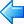Back to main directory:Software Practical